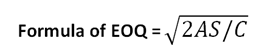222

## Latest \$type=blogging\$count=5\$author=hide\$comment=hide\$label=hide\$date=hide\$show=home

Economic Order Quantity Definition

Economic order quantity may be defined as that quantity of purchase which minimizes material order cost and material carrying cost. When purchase department orders for purchase, he thinks very deeply before such order. He is interested to reduce both order cost and carrying cost of material. Order cost is relating to the cost, if purchase department orders one time. Carrying cost is relating to the cost relating to rent of store and return on investment in stock and maintenance of stock. So, he will buy the material with economic way and use the economic order quantity formula for calculating the quantity where his cost will be minimized. The model and formula of EOQ was developed by F. Wilson Harris in 1913. He was very intelligent because he calculated optimal number of units of material, if we purchase that numbers of units, our total cost associated with purchase, delivery and storage will be minimum. EOQ is also known as re-order quantity.

A = Annual usage units or annual requirement or annual consumption of materials
S = Ordering cost per order

{ Note : Order cost per order is  a fixed cost because it does not affected with the quantity of material but it is charged on the process of transferring, it will be high with increasing the number of orders.}

C = Annual holding or carrying cost of one unit, (Carrying cost percentage X cost of one unit)

{ Note : In this cost, we can include interest on your capital, if you have borrowed money for buying the material, then interest on loan, insurance of material, taxes, storage cost }

These days, system of calculating above economic order quantity become automatically after coming of ERP softwares. But to know above formula manually is very necessary for clearing your basic fundamentals of inventory management.

Assumptions underlying EOQ

1. We can calculate ordering cost per order and carrying cost per unit per annual
2. We can also calculate estimated annual usage units of materials
3. Cost per unit of material is constant.
4. There is zero lead time means we can get material immediately.

For Example

Calculate the economic order quantity from the following information. Also state the number of orders to be placed in a year.

Consumption of material per year = 10,000 kgs

Order place cost per order = Rs. 50

Cost per kg of raw material = Rs. 2

Storage cost = 8% on average inventory or 8/100 X 2 = 0.16

EOQ = 2500 Kgs

No. of order to be placed in a year = 10000/2500 = 4 order per year

Benefits of Economic Order Quantity

1. Because, we are applying this formula and we are ready to buy the material at that total cost curve where is ordering cost and holding cost are equal, so we have to invest minimum amount for buying the material in different orders.

2. Because economic order quantity is also re-order quantity, so it can be used for calculating maximum level of stock.

How to Calculate EOQ PracticallyJust open ms excel sheet and calculate EOQ by writing different inputs of formula regarding to your manufacturing.

We used the following formula in Excel to calculate EOQ: =SQRT((2*B2*B3)/B4)

And the following formula to calculate Total Costs at this point: =\$B\$6/2*\$B\$4+\$B\$2/B6*\$B\$3

To create the graph, we used the following formulas and simply copied them over a range of 100 to 500 units.

Ordering Costs: =\$B\$2/D2*\$B\$3

Holding Costs: =D2/2*\$B\$4## 📚 Journal Entries eBook

🔥 Master Journal Entries Like a Pro!

Unlock Financial Success with our comprehensive Journal Entries eBook.

## 📘 Financial Accounting eBook

Enhance your financial knowledge with our Financial Accounting eBook.

## 🏢 Corporate Accounting eBook

Explore Corporate Accounting concepts with our eBook.

## 📑 Balance Sheet MADE Easy eBook

Simplify the Balance Sheet with our eBook.

## 📚 Online Accounting Course

Enhance your accounting skills with our online course.

Name

ltr
item
Accounting Education: Economic Order Quantity
Economic Order Quantity
http://2.bp.blogspot.com/_DJEIRrK4tl4/TF-tg3mFucI/AAAAAAAAF0c/184rEZmbMPg/s400/eoq.PNG
http://2.bp.blogspot.com/_DJEIRrK4tl4/TF-tg3mFucI/AAAAAAAAF0c/184rEZmbMPg/s72-c/eoq.PNG
Accounting Education
https://www.svtuition.org/2010/08/economic-order-quantity.html
https://www.svtuition.org/
https://www.svtuition.org/
https://www.svtuition.org/2010/08/economic-order-quantity.html
true
2410664366776677676
UTF-8